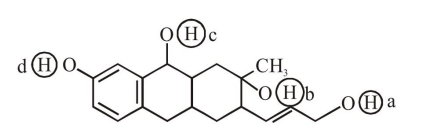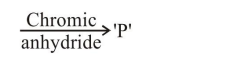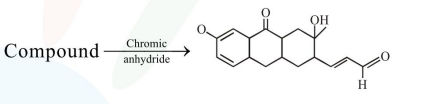# Consider the following reaction :

Question:

Consider the following reaction :The product 'P' gives positive ceric ammonium nitrate test. This is because of the presence of which of these $-\mathrm{OH}$ group $(\mathrm{s})$ ?

1. (c) and (d)

2. (b) only

3. (d) only

4. (b) and (d)

Correct Option: , 2

Solution:due to pressure of $\mathrm{b}$